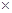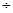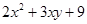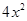1. /
2. CBSE
3. /
4. Class 08
5. /
6. Algebraic Expressions and Identities...

# Algebraic Expressions and Identities class 8 Notes Mathematics### myCBSEguide App

Download the app to get CBSE Sample Papers 2023-24, NCERT Solutions (Revised), Most Important Questions, Previous Year Question Bank, Mock Tests, and Detailed Notes.

Algebraic Expressions and Identities class 8 Notes Mathematics in PDF are available for free download in myCBSEguide mobile app. The best app for CBSE students now provides Algebraic Expressions and Identities class 8 Notes Mathematics latest chapter wise notes for quick preparation of CBSE exams and school-based annual examinations. Class 8 Mathematics notes on chapter 9 Algebraic Expressions and Identities Mathematics are also available for download in CBSE Guide website.

## Class 8 Mathematics notes Chapter 9 Algebraic Expressions and Identities

Download CBSE class 8th revision notes for chapter 9 Algebraic Expressions and Identities in PDF format for free. Download revision notes for Algebraic Expressions and Identities class 8 Notes and score high in exams. These are the Algebraic Expressions and Identities class 8 Notes prepared by team of expert teachers. The revision notes help you revise the whole chapter 9 in minutes. Revision notes in exam days is one of the best tips recommended by teachers during exam days.

## CBSE Class 8 Revision Notes Maths Algebraic Expressions and Identities

• Expressions are formed from variables and constants.
• Constant: A symbol having a fixed numerical value. Example: 2, , 2.1, etc.
• Variable: A symbol which takes various numerical values. Example: x, y, z, etc.
• Algebric Expression: A combination of constants and variables connected by the sign +, -,andis called algebraic expression.
• Terms are added to form expressions. Terms themselves are formed as product of factors.
• Expressions that contain exactly one, two and three terms are called monomials, binomials and trinomials respectively. In general, any expression containing one or more terms with non-zero coefficients (and with variables having non- negative exponents) is called a polynomial.
• Like terms are formed from the same variables and the powers of these variables are the same, too. Coefficients of like terms need not be the same.
• While adding (or subtracting) polynomials, first look for like terms and add (or subtract) them; then handle the, unlike terms.
• There are number of situations in which we need to multiply algebraic expressions: for example, in finding area of a rectangle, the sides of which are given as expressions.
• Monomial: An expression containing only one term. Example: -3, 4x, 3xy, etc.
• Binomial: An expression containing two terms. Example: 2x-3, 4x+3y, xy-4, etc.
• Trinomial: An expression containing three terms. Example:, 3x+2y+5z, etc.
• Polynomial: In general, any expression containing one or more terms with non-zero coefficients (and with variables having non-negative exponents). A polynomial may contain any number of terms, one or more than one.
• A monomial multiplied by a monomial always gives a monomial.
• While multiplying a polynomial by a monomial, we multiply every term in the polynomial by the monomial.
• In carrying out the multiplication of a polynomial by a binomial (or trinomial), we multiply term by term, i.e., every term of the polynomial is multiplied by every term in the binomial (or trinomial). Note that in such multiplication, we may get terms in the product which are like and have to be combined.
• An identity is an equality, which is true for all values of the variables in the equality. On the other hand, an equation is true only for certain values of its variables. An equation is not an identity.
• The following are the standard identities:
(a + b)2 = a2 + 2ab + b2 (I)
(a – b)2 = a2 – 2ab + b2 (II)
(a + b)(a – b) = a2 – b2 (III)
Another useful identity is (x + a) (x + b) = x2  + (a + b) x + ab  (IV)
• The above four identities are useful in carrying out squares and products of algebraic expressions. They also allow easy alternative methods to calculate products of numbers and so on.
• Coefficients: In the term of an expression any of the factors with the sign of the term is called the coefficient of the product of the other factors.
• Terms: Various parts of an algebraic expression which are separated by + and – signs. Example: The expression 4x + 5 has two terms 4x and 5.
(i)  Constant Term: A term of expression having no lateral factor.
(ii)  Like term: The term having the same literal factors. Example 2xy and -4xy are like terms.
(iii)  Unlike term: The terms having different literal factors. Example:and 3xy are unlike terms.
• Factors: Each term in an algebraic expression is a product of one or more number (s) and/or literals. These number (s) and/or literal (s) are known as the factor of that term. A constant factor is called numerical factor, while a variable factor is known as a literal factor. The term 4x is the product of its factors 4 and x.

## CBSE Class 8 Revision Notes and Key Points

Algebraic Expressions and Identities class 8 Notes Mathematics. CBSE quick revision note for class-8 Mathematics, Chemistry, Math’s, Biology and other subject are very helpful to revise the whole syllabus during exam days. The revision notes covers all important formulas and concepts given in the chapter. Even if you wish to have an overview of a chapter, quick revision notes are here to do if for you. These notes will certainly save your time during stressful exam days.

To download Algebraic Expressions and Identities class 8 Notes Mathematics, sample paper for class 8 Mathematics, Social Science, Science, Mathematics; do check myCBSEguide app or website. myCBSEguide provides sample papers with solution, test papers for chapter-wise practice, NCERT solutions, NCERT Exemplar solutions, quick revision notes for ready reference, CBSE guess papers and CBSE important question papers. Sample Paper all are made available through the best app for CBSE students and myCBSEguide website.### Test Generator

Create question paper PDF and online tests with your own name & logo in minutes.### myCBSEguide

Question Bank, Mock Tests, Exam Papers, NCERT Solutions, Sample Papers, Notes